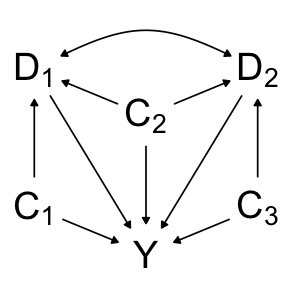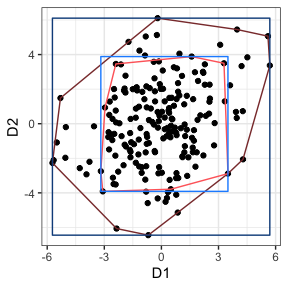# Multivariate generalized propensity score: An introduction

#### 2021-12-06

The goal of this package is to expand currently available software to estimate weights for multivariate continuous exposures. Weights are formed assuming a multivariate normal distribution for the simultaneous exposures.

# Installation

You can install mvGPS from GitHub using the following code:

install.packages("devtools")
devtools::install_github("williazo/mvGPS")

## Example

### Data Generating

To illustrate a simple setting where this multivariate generalized propensity score would be useful, we can construct a directed acyclic graph (DAG) with a bivariate exposure, D=(D1, D2), confounded by a set C=(C1, C2, C3). In this case we assume C1 and C2 are associated with D1, while C2 and C3 are associated with D2 as shown below.To generate this data we first draw n=200 samples from C assuming a multivariate normal distribution with mean equal to zero, variance equal to 1, and constant covariance of 0.1.

Next we define our exposure as a linear function of our confounders. Explicitly these two equations are defined as

E[D1|C]=0.5C1+C2,

E[D2|C]=0.3C2+0.75C3.

With this construction, the exposures have one confounder in common, C2, and one independent confounder. The effect size of the confounders vary for each exposure. We assume that the conditional distribution of D given C is bivariate normal with conditional correlation equal to 0.2 and conditional variance equal to 2.

To generate the set of confounders and the corresponding bivariate exposure we can use the function gen_D() as shown below.

require(mvGPS)
sim_dt <- gen_D(method="u", n=200, rho_cond=0.2, s_d1_cond=2, s_d2_cond=2, k=3,
C_mu=rep(0, 3), C_cov=0.1, C_var=1,
d1_beta=c(0.5, 1, 0), d2_beta=c(0, 0.3, 0.75), seed=06112020)
D <- sim_dt$D C <- sim_dt$C

By construction our marginal correlation of D is a function of parameters from the distribution of C, coefficients of conditional mean equations, and conditional covariance parameter. For the above specification the true marginal correlation of exposure is equal to 0.24 and our observed marginal correlation is equal to 0.26.

Finally, we specify our outcome, Y, as a linear combination of the confounders and exposure. The mean of the dose-response equation is shown below,

E[Y|D, C]=0.75C1+1C2+0.6C3+D1+D2.

Both exposures have treatment effect sizes equal to one. The standard deviation of our outcome is set equal 2.

alpha <- c(0.75, 1, 0.6, 1, 1)
sd_Y <- 2
X <- cbind(C, D)
Y <- X%*%alpha + rnorm(200, sd=sd_Y)

### Generating Weights

With the data generated, we can now use our primary function mvGPS() to estimate weights. These weights are constructed such that the numerator is equal to the marginal density, with the denominator corresponding to the conditional density, i.e., the multivariate generalized propensity score.

w = f(D)/f(D|C)

In our case since the bivariate exposure is assumed to be bivariate normal, we can break both the numerator and denominator into full conditional densities knowing that each univariate conditional expression will remain normally distributed.

w = f(D2|D1)f(D1)/f(D2|D1,C2,C3)f(D1|C1,C2)

Notice in the equation above, we are also able to specify the confounding set for each exposure separately.

require(mvGPS)
out_mvGPS <- mvGPS(D=D, C=list(C[, 1:2], C[, 2:3]))
w <- out_mvGPS$w This vector w now can be used to test balance of confounders by comparing weighted vs. unweighted correlations and to estimate the treatment effects using weighted least squares regression. ### Balance Assessment For continuous exposure(s) we can asses balance using several metrics such as euclidean distance, maximum absolute correlation, and average absolute correlation where correlation refers to the Pearson correlation between exposure and covariate. Below we use the function bal() to specify a set of potential models to use for comparison. Possible models that are available include: mvGPS, Entropy, CBPS, GBM, and PS. For methods other than mvGPS which can only estimate univariate continuous exposure, each exposure is fit separately so that weights are generated for both exposures. require(knitr) bal_results <- bal(model_list=c("mvGPS", "entropy", "CBPS", "PS", "GBM"), D, C=list(C[, 1:2], C[, 2:3])) bal_summary <- bal_results$bal_metrics
#contains overall summary statistics with respect to balance
bal_summary <-data.frame(bal_summary, ESS=c(bal_results$ess, nrow(D))) #adding in ESS with last value representing the unweighted case bal_summary <- bal_summary[order(bal_summary$max_cor), ]

kable(bal_summary[, c("euc_dist", "max_cor", "avg_cor", "ESS", "method")],
digits=4, row.names=FALSE,
col.names=c("Euc. Distance", "Max. Abs. Corr.",
"Avg. Abs. Corr.", "ESS", "Method"))
Euc. Distance Max. Abs. Corr. Avg. Abs. Corr. ESS Method
0.0930 0.0884 0.0331 163.8253 mvGPS
0.2568 0.2145 0.1137 159.9284 GBM_D1
0.2592 0.2288 0.1085 179.8179 PS_D1
0.3095 0.2321 0.1092 178.8683 entropy_D2
0.2400 0.2336 0.0721 172.2635 entropy_D1
0.2843 0.2400 0.1236 184.3871 CBPS_D1
0.3185 0.2418 0.1227 180.3586 PS_D2
0.3285 0.2502 0.1219 142.8799 GBM_D2
0.5009 0.3168 0.2421 200.0000 unweighted
1.1022 0.6701 0.5012 50.2385 CBPS_D2

We can see that our method mvGPS achieves the best balance across both exposure dimensions. In this case we can also note that the effective sample size after weighting 163.8253 is still sufficiently large that we not worried about loss of power.

### Bias Reduction

Finally, we want to check that these weights are properly reducing the bias when we estimate the exposure treatment effect.

dt <- data.frame(Y, D)
mvGPS_mod <- lm(Y ~ D1 + D2, weights=w, data=dt)
mvGPS_hat <- coef(mvGPS_mod)[c("D1", "D2")]

unadj_hat <- coef(lm(Y ~ D1 + D2, data=dt))[c("D1", "D2")]

kable(bias_tbl, digits=2, row.names=TRUE,
col.names=c("Truth", "Unadjusted", "mvGPS"))
D1 1 1.28 1.12
D2 1 1.18 1.05

To compare the total reduction at bias we look at the total absolute bias where we see mvGPS has total bias equal to 0.18, or an average percent bias of 8.79% per exposure, compared to unadjusted total bias equal to 0.45, or an average percent bias of 22.62% per exposure. We therefore achieve 2.57 times reduction in bias.

### Defining Estimable Region

An important consideration when using propensity scores to estimate causal effects are the three key identifying assumptions:

1. weak ignorability, aka, unconfoundedness, aka, selection on observables
2. stable unit treatment value (SUTVA)
3. positivity

Weak ignorability assumes that the exposure is conditionally independent of the potential outcomes given the appropriate set of confounders. Checking balance as shown above is one of the key diagnostics to determining the legitimacy of this assumption in practice.

SUTVA states that the potential outcome of each unit does not depend on the exposure that other units receive and that there exists only one version of each exposure. It is generally an untestable assumption, but is key to ensuring that the potential outcomes are well-defined and that the observed outcome given the observed exposure corresponds to the true potential outcome.

The final identifying assumption, positivity, is our focus when defining estimable regions for multivariate exposure. Positivity posits that all units have the potential to receive a particular level of exposure given any value of the confounders. The upshot of this is that we need to take care when defining the domain of our exposure when estimating the mvGPS.

Typically in the case of univariate continuous exposure, we often ensure positivity by restricting the domain to the observed range of exposure or a trimmed version. A logical extension to the multivariate exposure would be to define our domain as the product of the range of each exposure. However, when the exposures of interest are correlated this domain may not be appropriate. Recall that in our simulated data the marginal correlation of D1 and D2 is 0.26.

Instead, we propose to ensure positivity with multivariate exposures by defining the domain as the multidimensional convex hull of the observed exposure values. To obtain the convex hull of our exposure we use the function hull_sample(). This will return the vertices of the convex hull, and in the case of bivariate exposure it will also sample equally along a grid of the convex hull and return these values which can be used for calculating the dose-response surface.

Note that we can also create trimmed versions of either the product of ranges or convex hull as shown below.

require(sp)
require(ggplot2)
chull_D <- hull_sample(D)
#generate convex hull of exposure
chull_D_trim <- hull_sample(D, trim_hull=TRUE, trim_quantile=0.95)
#generate trimmed convex hull

bbox_grid <- sp::bbox(chull_D$hpts_vs) #bounding box over convex hull bbox_df <- data.frame(D1=c(bbox_grid[1, 1], bbox_grid[1, 2], bbox_grid[1, 2], bbox_grid[1, 1]), D2=c(bbox_grid[2, 1], bbox_grid[2, 1], bbox_grid[2, 2], bbox_grid[2, 2])) bbox_grid_trim <- sp::bbox(chull_D_trim$hpts_vs) #bounding box over trimmed convex hull
bbox_df_trim <- data.frame(D1=c(bbox_grid_trim[1, 1], bbox_grid_trim[1, 2],
bbox_grid_trim[1, 2], bbox_grid_trim[1, 1]),
D2=c(bbox_grid_trim[2, 1], bbox_grid_trim[2, 1],
bbox_grid_trim[2, 2], bbox_grid_trim[2, 2]))
chull_plot <- ggplot(data=data.frame(D), aes(x=D1, D2))+
geom_point()+
geom_polygon(data=data.frame(chull_D$hpts_vs), color="indianred4", fill=NA)+ geom_polygon(data=data.frame(chull_D_trim$hpts_vs), color="indianred1", fill=NA, alpha=0.4)+
geom_polygon(data=bbox_df, color="dodgerblue4", fill=NA)+
geom_polygon(data=bbox_df_trim, color="dodgerblue1", fill=NA, alpha=0.4)+
xlab("D1")+
ylab("D2")+
theme_bw()
chull_plotIn dark red we have the observed convex hull and in light red we have the trimmed convex hull at the 95th percentile. In dark blue we have the observed product range and in light blue we have the trimmed product range at the 95th percentile.

Notice that by trimming we are further restricting our domains to high density regions of the exposure. We can also see that by restricting to the convex hull we are avoiding areas with sparse data that are included in the product range domain.

### Dose-Response Surface

When exposure is bivariate, the resulting dose-response function is a surface. Using the weighted regression model described above to incorporate the weights, we can predict across our convex hull domain to gain intuition about how altering the exposures effects the outcome of interest. To see an example of this type of dose-response surface on an application to analyzing obesity intervention programs in Los Angeles County visit https://williazo.github.io/resources/.